# How to Calculate and Solve for Loss on Ignition | Refractories

The loss on ignition is illustrated by the image below.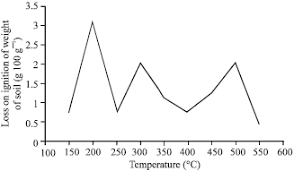To compute for loss on ignition, two essential parameters are needed and these parameters are Weight before Firing (m1) and Weight after Firing (m2).

The formula for calculating loss on ignition:

LOI = 100((m1 – m2)/m1)

Where:

LOI = Loss On Ignition
m1 = Weight before Firing
m2 = Weight after Firing

Let’s solve an example;
Find the loss on ignition when the weight before firing is 22 and the weight after firing is 11.

This implies that;

m1 = Weight before Firing = 22
m2 = Weight after Firing = 11

LOI = 100((m1 – m2)/m1)
LOI = 100((22 – 11)/22)
LOI = 100(11/22)
LOI = 100(0.5)
LOI = 50

Therefore, the loss on ignition is 50%.

Nickzom Calculator – The Calculator Encyclopedia is capable of calculating the loss on ignition.

To get the answer and workings of the loss on ignition using the Nickzom Calculator – The Calculator Encyclopedia. First, you need to obtain the app.

You can get this app via any of these means:

You can also try the demo version via https://www.nickzom.org/calculator

Apple (Paid) – https://itunes.apple.com/us/app/nickzom-calculator/id1331162702?mt=8
Once, you have obtained the calculator encyclopedia app, proceed to the Calculator Map, then click on Materials and Metallurgical under Engineering.Now, Click on Refractories under Materials and Metallurgical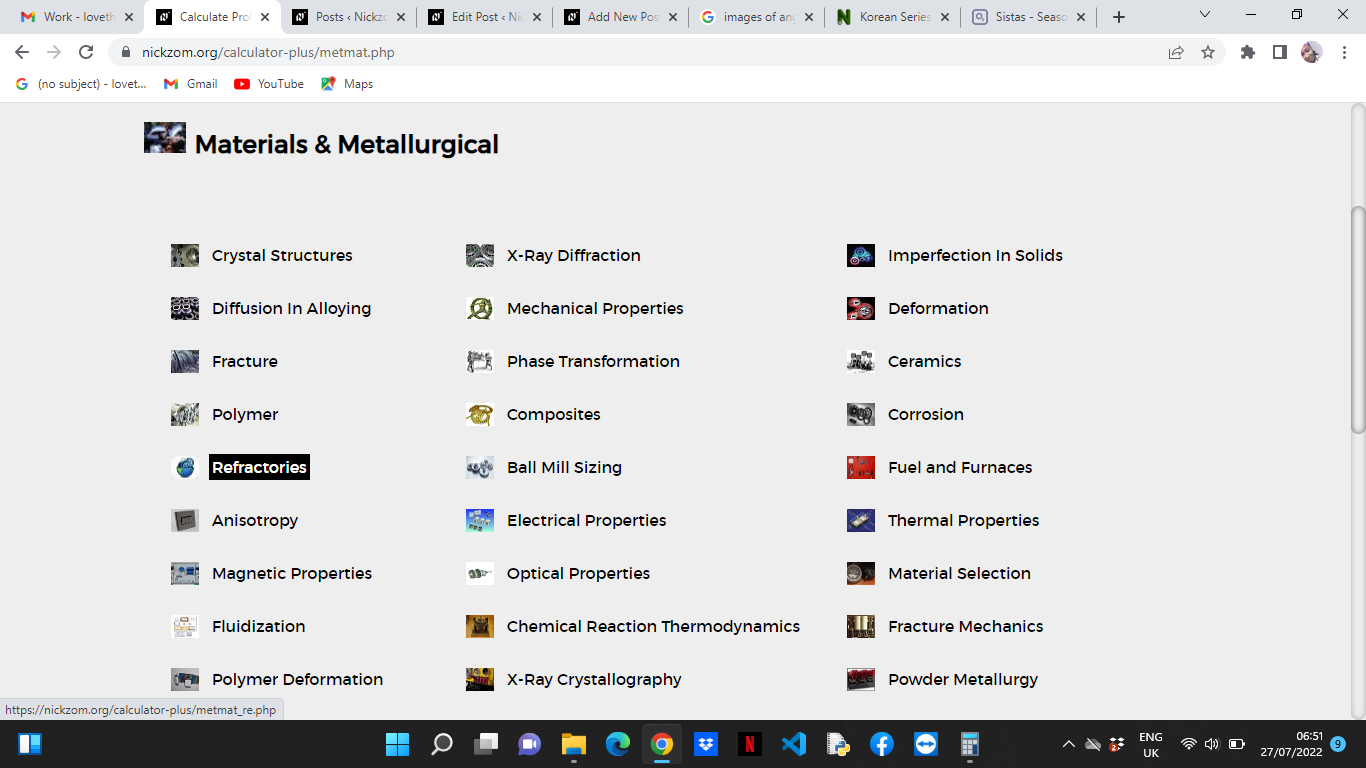Now, Click on Loss on Ignition under Refractories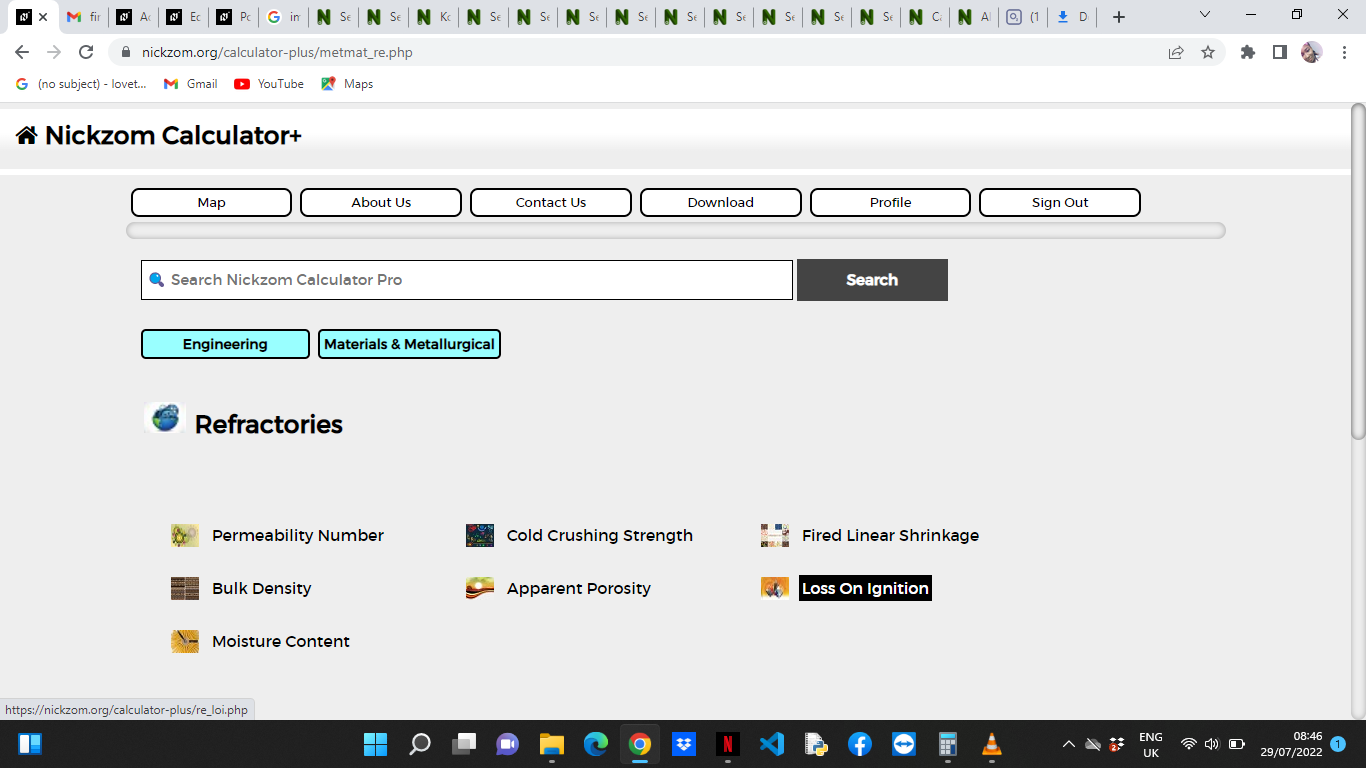The screenshot below displays the page or activity to enter your values, to get the answer for the loss on ignition according to the respective parameter which is the Weight before Firing (m1) and Weight after Firing (m2).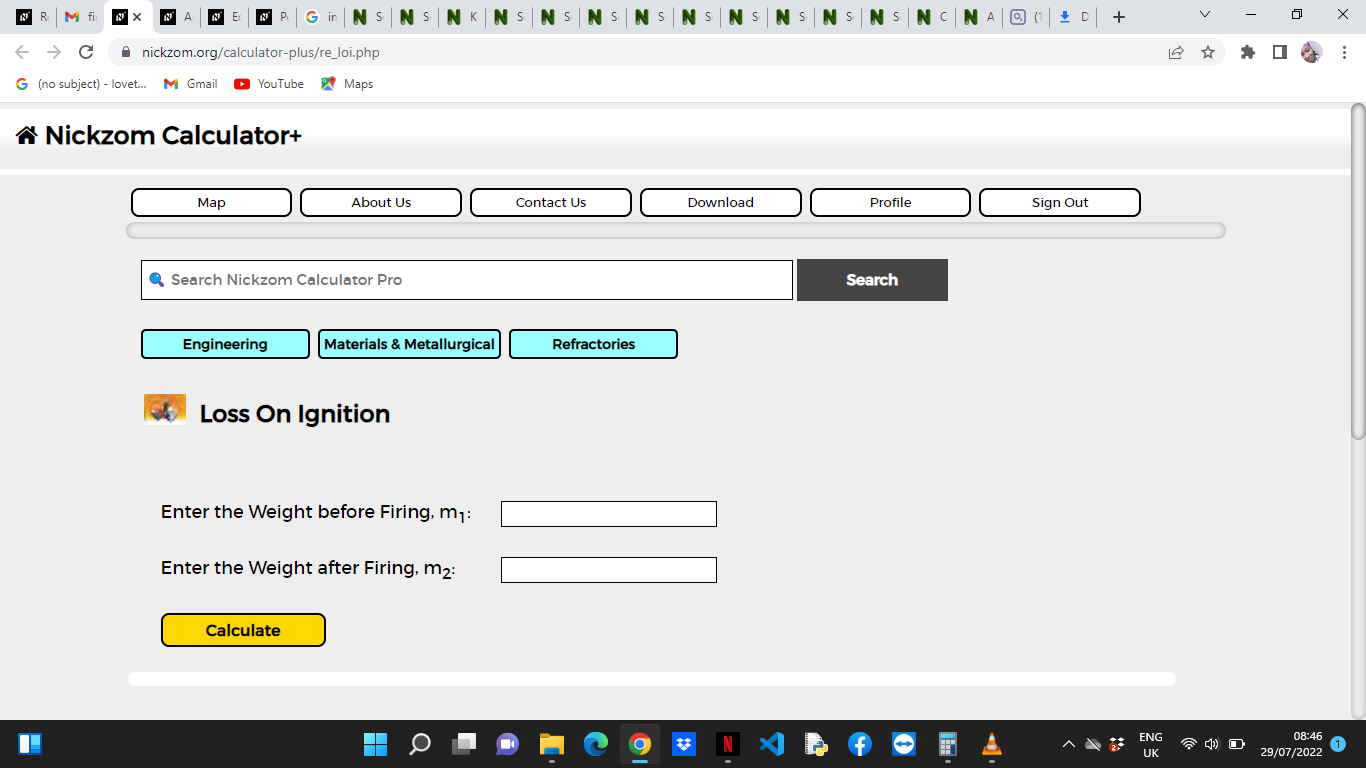Now, enter the values appropriately and accordingly for the parameters as required by the Weight before Firing (m1is 22 and Weight after Firing (m2) is 11.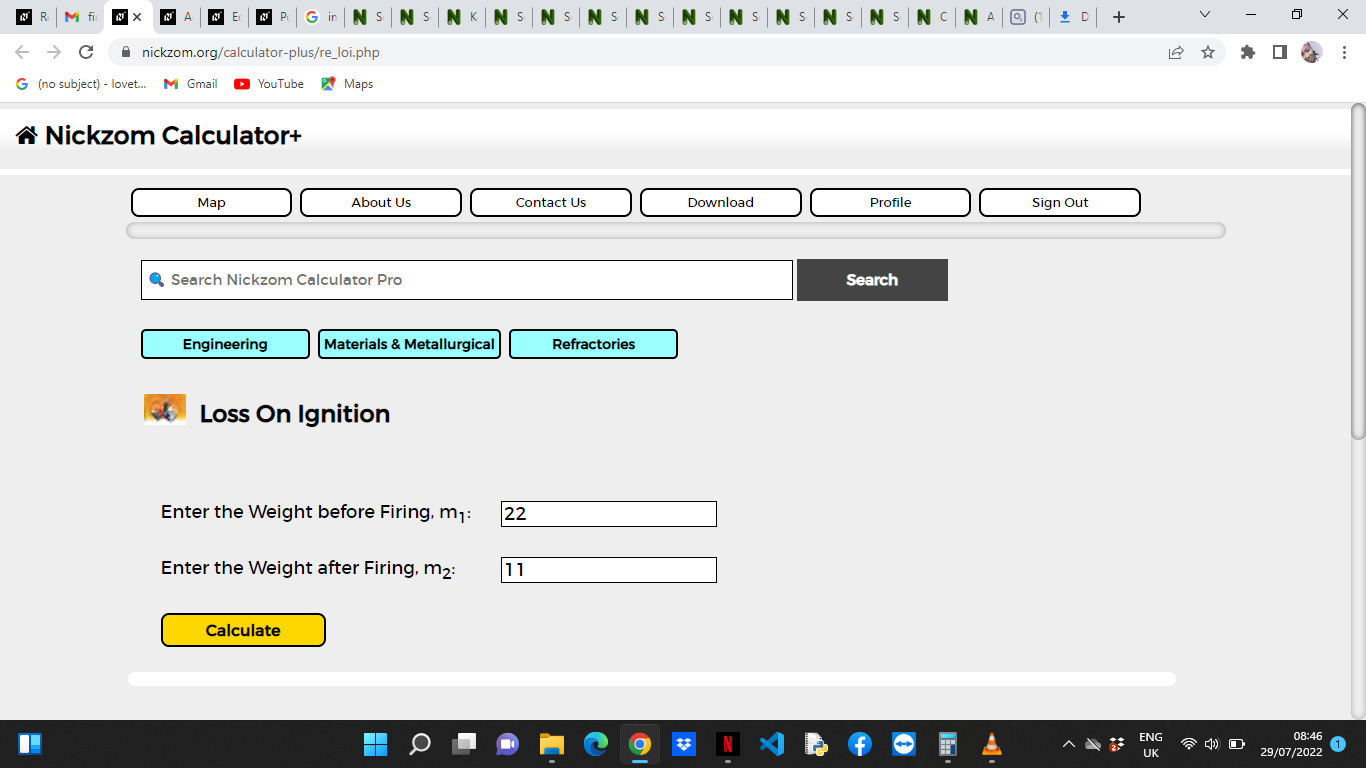Finally, Click on Calculate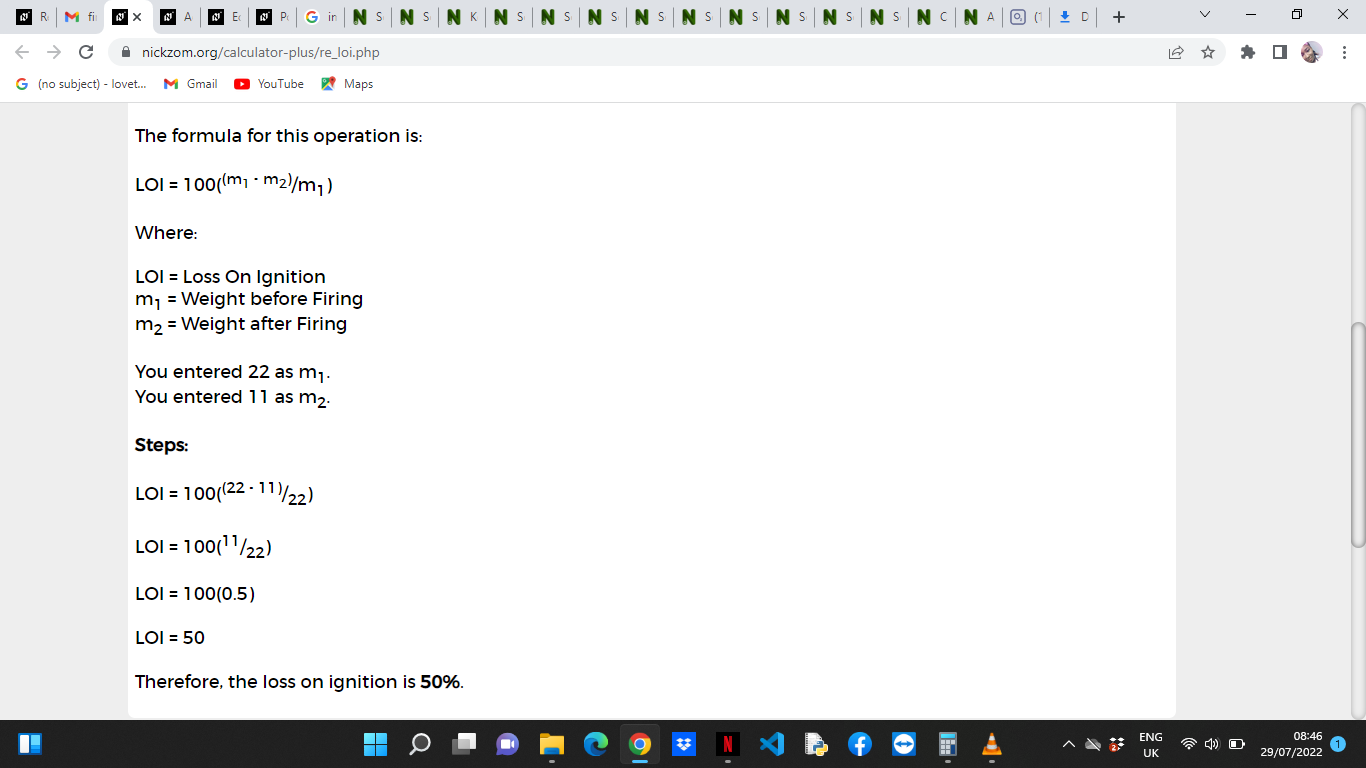As you can see from the screenshot above, Nickzom Calculator– The Calculator Encyclopedia solves for the loss on ignition and presents the formula, workings and steps too.